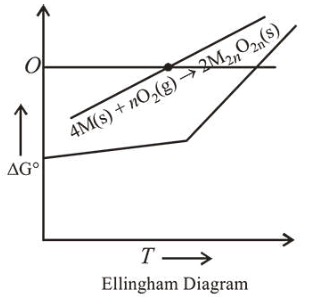# For a reaction,Question:

For a reaction, $4 \mathrm{M}(\mathrm{s})+\mathrm{nO}_{2}(\mathrm{~g}) \rightarrow 2 \mathrm{M}_{2} \mathrm{O}_{\mathrm{n}}(\mathrm{s})$, the free energy change is plotted as a function of temperature. The temperature below which the oxide is stable could be inferred from the plot as the point at which :

1. The slope change from negative to positive

2. The free energy change shows a change from negative to positive value

3. The slop changes from positive to negative

4. The slop changes from positive to zero

Correct Option: , 2

Solution:From graph it is evident that the temperature below which the oxide is stable, is the point at which free energy change shows a change from negative to positive.